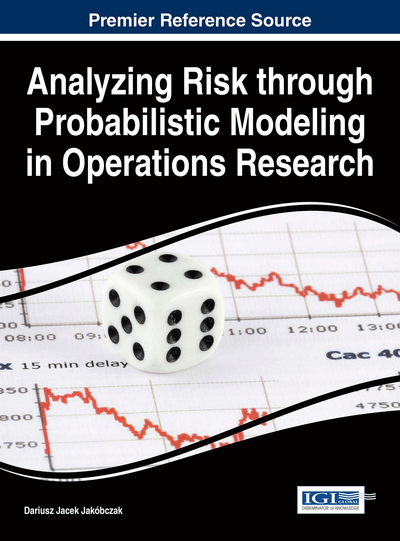# Perfect Partners of Mathematical Modeling with Technology in Risk Assessment

William P. Fox (Naval Postgraduate School, USA)
DOI: 10.4018/978-1-4666-9458-3.ch003

## Abstract

This chapter discusses the use of mathematical modeling with technology in risk assessment in the broad area of operations research. The authors provide modeling as a process and illustrate suggested steps in the process. This chapter reviews some of the main modeling texts and provide a brief discussion of their processes. Many illustrative examples are provided to show the breadth of mathematical modeling. These examples cover such topics as discrete dynamical systems, game theory, multi-attribute decision making, data envelopment analysis with linear programming, and integer programming. The authors discuss the important of sensitivity analysis, as applicable. Several scenarios are used as illustrative examples of the process.
Chapter Preview
Top

## Introduction

Consider the importance of modeling for decision making in business (B), industry (I), and government (G). BIG decision making is essential to success at all levels. We do not encourage “shooting from the hip”. We recommend good analysis for the decision maker to examine and question in order to find the best alternative to choose or decision to make. This book explains the modeling process and provides examples of decision making throughout.

Let’s describe a mathematical model as a mathematical description of a system using the language of mathematics. The process of developing such a mathematical model is termed mathematical modeling. Mathematical models are used in the natural sciences (such as physics, biology, earth science, meteorology), engineering disciplines (e.g. computer science, systems engineering, operations research, and in the social sciences (such as business, economics, psychology, sociology, political science, and social networks). The professional is these areas use mathematical models all the time. A mathematical model may be used to help explain a system, to study the effects of different components, or to make predictions about behavior (Giordano, Fox, & Horton, 2013).

Mathematical models can take many forms, including but not limited to dynamical systems, statistical models, regression models, differential equations, optimization models, or game theoretic models. These and other types of models can overlap, or one output becomes the input for another similar or different model form. In many cases, the quality of a scientific field depends on how well the mathematical models developed on the theoretical side agree with results of repeatable experiments (Giordano, et al, 2013). Any lack of agreement between theoretical mathematical models and experimental measurements could lead to model refinements and better, more useful, models. We do not plan to cover all the mathematical modeling processes here. We only provide an overview to the decision makers. Our goal is to offer competent, confident problem solvers for the 21st century. We suggest the references listed at the end of this chapter in order to get more familiar with the many techniques in mathematical modeling.

## Complete Chapter List

Search this Book:
Reset